# Obtain and Fit a Radial Profile## Overview

#### Synopsis:

The surface brightness flux is determined by finding the net counts in a stack of concentric annuli and then dividing by the respective areas. A specified analytic model may be fit to the resultant histogram. This information can be used, for instance, to provide evidence for extended emission and calculate the hardness ratio thereof.

#### Purpose:

To produce radial profiles of an HRC or ACIS imaging observation.

Last Update: 7 Feb 2022 - Review for CIAO 4.14. Updated for Repro-5 and CALDB 4.9.6.

## Get Started

`unix% download_chandra_obsid 1838 evt2`

In the following examples, restrict the energy range of the events:

```unix% dmcopy "acisf01838N003_evt2.fits[energy=300:8000]" acis_1838_evt2.fits
```

The ability of dmextract to operate on a stack of regions makes it possible to compute radial profiles simply by defining multiple concentric annuli.

### 1. Creating Multiple Annuli

Display the file:

```unix% ds9 acis_1838_evt2.fits &
```

Select Region → Shape → Annulus and left-click on the image. A singular annular region will appear. To edit the region, make it active (left-click) and select "Get Info..." from the Region menu.

A region editing window (Figure 1) will appear, in which one can adjust the number of annuli and their sizes. Thirty-eight equally-spaced annuli, with minimium and maximum of 10 and 200 pixels respectively, which are located around (but exclude) the core of G21.5-09, are shown in Figure 2.

### Figure 1: Annulus editing window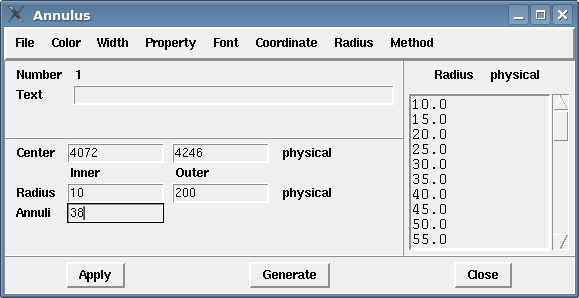### Figure 1: Annulus editing window

The parameters are set to create 38 equally-spaced annuli centered at (4062,4240).

### Figure 2: Source annuli overlaid on data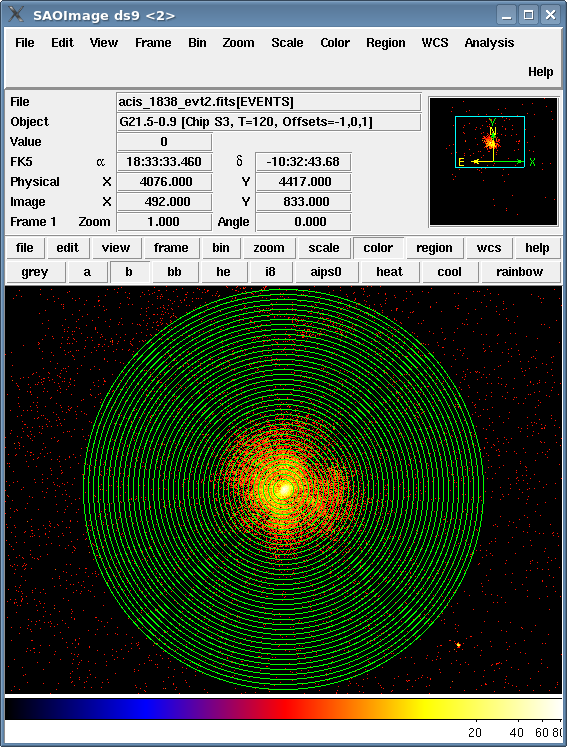### Figure 2: Source annuli overlaid on data

The minimum radius is 10 pixels and the maximum radius is 200 pixels.

Save the annuli:

1. Region → Save Regions... → Save As "annuli.reg".
2. After choosing "OK" in the region filename dialog, a format dialog is opened. Set the format to "CIAO" and the coordinate system to "Physical".

Follow similar steps to create a a background annulus (Figure 3) from 200 to 225 pixels. The background is saved as "annuli_bgd.reg".

### Figure 3: Background annulus overlaid on data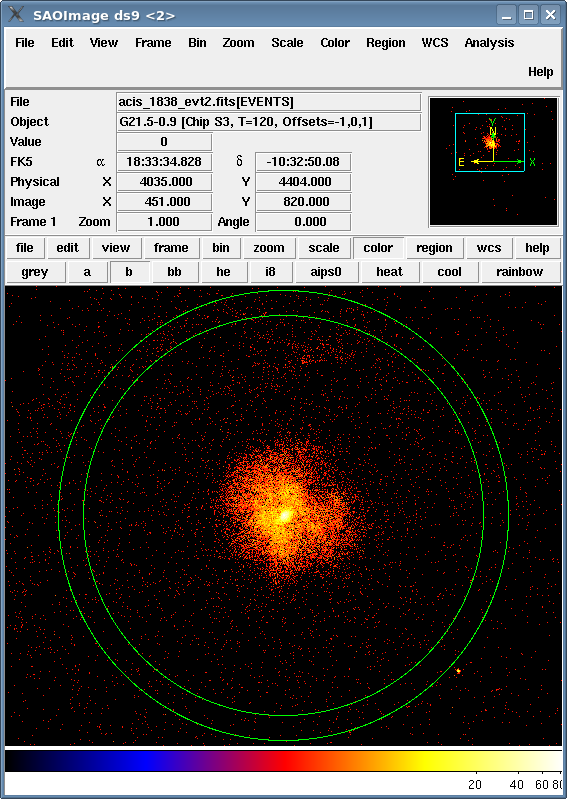### Figure 3: Background annulus overlaid on data

The background region has been defined as an annulus with inner radius of 200 pixels and outer radius of 225 pixels.

The source region file looks like this:

```unix% more annuli.reg
# Region file format: CIAO version 1.0
annulus(4062,4240,10,15)
annulus(4062,4240,15,20)
annulus(4062,4240,20,25)
.
. (etc.)
.
annulus(4062,4240,190,195)
annulus(4062,4240,195,200)
```

and the background annulus like this:

```unix% more annuli_bgd.reg
# Region file format: CIAO version 1.0
annulus(4062,4240,200,225)
```

### 2. Removing Contaminating Point Sources

Suppose that the annuli had a maximum radius of 250 pixels in the previous step. The point source circled in green in Figure 4 would then contribute to a few of the radial profiles.

### Figure 4: Annuli that contain an unwanted point source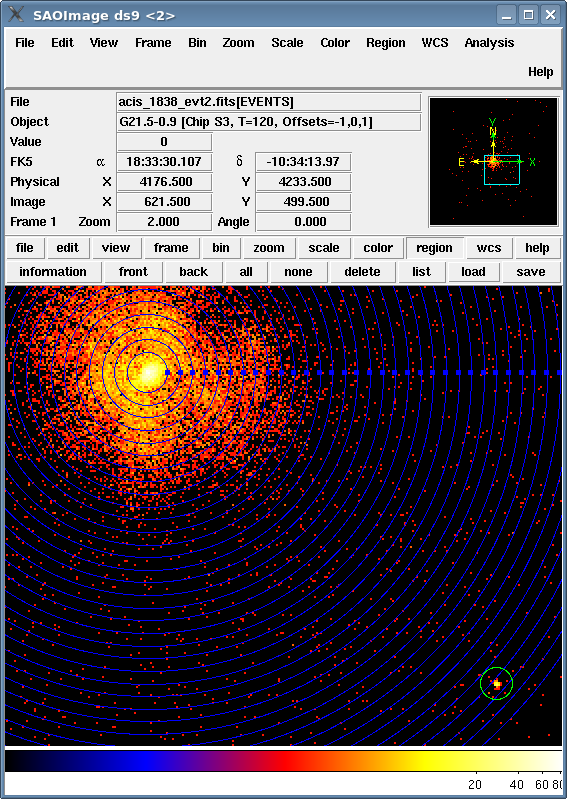### Figure 4: Annuli that contain an unwanted point source

The green circle shows the source that needs to be removed.

Having saved the region in ds9:

```unix% more contam.reg
# Region file format: CIAO version 1.0
circle(4235.9495,4084.4343,8)
```

it is easy to remove this point source before generating the radial profiles:

```unix% dmcopy "acis_1838_evt2.fits[exclude sky=region(contam.reg)]" acis_1838_excl_evt2.fits
```

This command creates a new event file with the point source removed (Figure 5). Use this event file in the rest of the radial profile analysis. This is not an issue in this example, so we continue using acis_1838_evt2.fits.

### Figure 5: Event file with source removed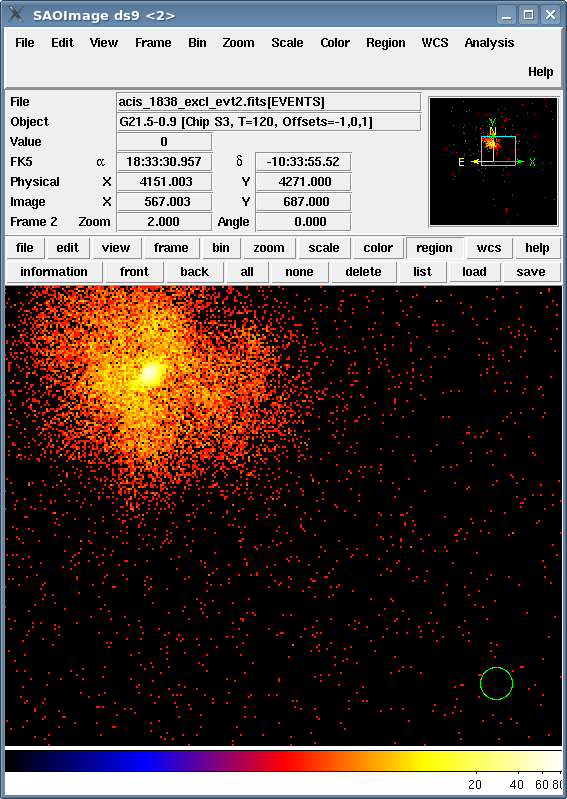### Figure 5: Event file with source removed

The green circle shows where the unwanted source used to be located.

### 3. Run dmextract

It is now possible to run dmextract to extract the radial profiles:

```unix% punlearn dmextract
unix% pset dmextract infile="acis_1838_evt2.fits[bin sky=@annuli.reg]"
unix% pset dmextract outfile=1838_rprofile.fits
unix% pset dmextract bkg="acis_1838_evt2.fits[bin sky=@annuli_bgd.reg]"
unix% pset dmextract opt=generic
unix% dmextract
Input event file  (acis_1838_evt2.fits[bin sky=@annuli.reg]):
Enter output file name (1838_rprofile.fits):
```

The contents of the parameter file may be checked using plist dmextract.

The tool calculates several new columns, the surface brightness (SUR_BRI) and its error (SUR_BRI_ERR) among them:

```unix% dmlist 1838_rprofile.fits cols

--------------------------------------------------------------------------------
Columns for Table Block HISTOGRAM
--------------------------------------------------------------------------------

ColNo  Name                 Unit        Type             Range
.
. (output omitted)
.
20   NET_COUNTS           count        Real8          -Inf:+Inf            Net Counts
21   NET_ERR              count        Real8          -Inf:+Inf            Error on Net Counts
22   NET_RATE             count/s      Real8          -Inf:+Inf            Net Count Rate
23   ERR_RATE             count/s      Real8          -Inf:+Inf            Error Rate
24   SUR_BRI              count/pixel**2 Real8          -Inf:+Inf            Net Counts per square pixel
25   SUR_BRI_ERR          count/pixel**2 Real8          -Inf:+Inf            Error on net counts per square pixel
.
.
.
```

SUR_BRI is calculated as NET_COUNTS/AREA (columns 19 and 7, respectively); SUR_BRI_ERR is NET_ERR/AREA (columns 20 and 7).

Note that since the surface brightness is calculated from the NET_COUNTS column, the background counts are already removed from it: NET_COUNTS = COUNTS - [(BG_COUNTS/BG_AREA) * AREA]. It is therefore not necessary to account for the background separately when fitting this data.Profile in Flux Units

Suppose you are interested in a radial profile in units of flux (photons/cm2/pix2/s or photons/cm2/arcsec2/s). Exposure-corrected images (e.g. the "flux" image file produced by fluximage and merge_obs) should NOT be used; while the surface brightness profile will be correctly determined, the uncertainties will be incorrect. Instead, in order to appropriately propagate the errors with dmextract, an input image in units of counts (or an input energy-filtered event file) should be used, and in addition an exposure map supplied to provide the counts-to-flux conversion.

```unix% fluximage infile=acis_1838_evt2.fits outroot=1838 bands="0.3:8.0:2.5" binsize=1

unix% dmextract infile="1838_0.3-8.0_thresh.img[bin sky=@annuli.reg]" \
? outfile=1838_flux_rprofile.fits \
? bkg="1838_0.3-8.0_thresh.img[bin sky=@annuli_bgd.reg]" \
? exp=1838_0.3-8.0_thresh.expmap \
? bkgexp= 1838_0.3-8.0_thresh.expmap \
? opt=generic

— or extracting from an events file:

unix% dmextract infile="acis_1838_evt2.fits[energy=300:8000][bin sky=@annuli.reg]" \
? outfile=1838_flux_rprofile.fits \
? bkg="acis_1838_evt2.fits[energy=300:8000][bin sky=@annuli_bgd.reg]" \
? exp=1838_0.3-8.0_thresh.expmap \
? bkgexp= 1838_0.3-8.0_thresh.expmap \
? opt=generic
```

The tool calculates several new columns, including the surface brightness in flux units, (SUR_FLUX) and its error (SUR_FLUX_ERR), where the surface brightness is calculated from the NET_FLUX column with background accounted for: NET_FLUX = FLUX - (AREA / BG_AREA) * BG_COUNTS / (EXPOSURE * MEAN_BG_EXP).

The CEL_FLUX and CEL_FLUX_ERR columns are also provided, with transforms applied to convert values in pixels to ones in arcsec (if the appropriate WCS information is provided in the input file).New in CIAO 4.11

dmextract in CIAO 4.11 now creates the RMID column. It is the average of the annulus radii.

```unix% dmlist 1838_rprofile.fits'[cols R,RMID]' data

--------------------------------------------------------------------------------
Data for Table Block HISTOGRAM
--------------------------------------------------------------------------------

ROW    R                                     RMID

1                [       10.0        15.0]                12.50
2                [       15.0        20.0]                17.50
3                [       20.0        25.0]                22.50
4                [       25.0        30.0]                27.50
5                [       30.0        35.0]                32.50
...
```

## Plotting and Fitting

The radial profile can now be plotted in python using matplotlib

```unix% python

>>> import matplotlib.pylab as plt
>>>
>>> xx = tab.get_column("rmid").values
>>> yy = tab.get_column("sur_bri").values
>>> ye = tab.get_column("sur_bri_err").values
>>>
>>> plt.errorbar(xx,yy,yerr=ye, marker="o")
>>> plt.xscale("log")
>>> plt.yscale("log")
>>> plt.xlabel("R_MID (pixel)")
>>> plt.ylabel("SUR_BRI (photons/cm**2/pixel**2/s)")
>>> plt.title('G21.5-0.9 [Chip S3, T=120, Offsets=-1,0,1]')
```

which produces Figure 6.

### Figure 6: Radial profile of the source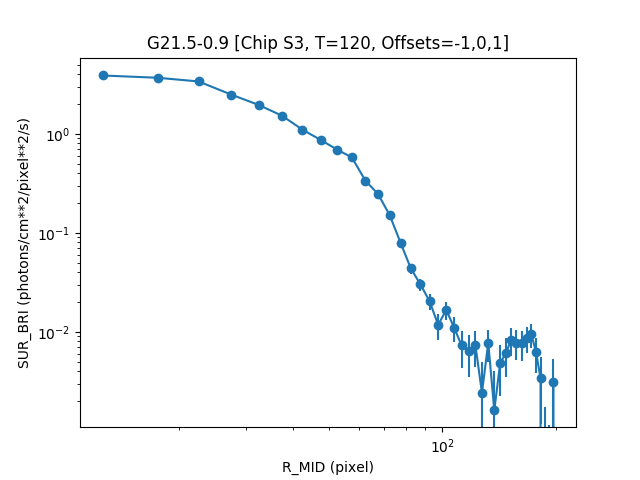### Figure 6: Radial profile of the source

A model can be fit to the measured surface brightness profile using Sherpa. As mentioned before, the background counts are already removed from the surface brightness, so it is not necessary to account for the background separately when fitting the data.

```unix% sherpa

sherpa> set_source("beta1d.src")
sherpa> src.r0 = 105
sherpa> src.beta = 4
sherpa> src.ampl = 0.00993448

sherpa> freeze(src.xpos)

sherpa> fit()
Dataset               = 1
Method                = levmar
Statistic             = chi2
Initial fit statistic = 4.24792e+11
Final fit statistic   = 221.594 at function evaluation 30
Data points           = 38
Degrees of freedom    = 35
Probability [Q-value] = 5.65854e-29
Reduced statistic     = 6.33127
Change in statistic   = 4.24792e+11
src.r0         134.119      +/- 0
src.beta       4.6542       +/- 0.0351998
src.ampl       1.51907e-06  +/- 1.70734e-08

sherpa> plot_fit()
sherpa> import matplotlib.pylab as plt
sherpa> plt.xscale("log")
sherpa> plt.yscale("log")
```

which produces Figure 7.

### Figure 7: Fit to the radial profile of the source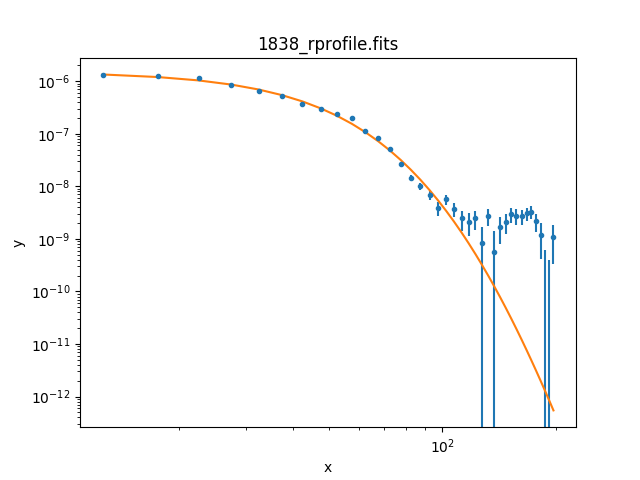### Figure 7: Fit to the radial profile of the source

Note that the effects of 2D blurring in a 2D image cannot be reproduced by convolving the radial profile of the PSF with a profile of the model. See "Accounting for PSF Effects in 2D Image Fitting".

```

#--------------------------------------------------------------------
#
# DMEXTRACT -- extract columns or counts from an event list
#
#--------------------------------------------------------------------
infile = acis_1838_evt2.fits[bin sky=@annuli.reg] Input event file
outfile = 1838_rprofile.fits    Enter output file name
(bkg = acis_1838_evt2.fits[bin sky=@annuli_bgd.reg]) Background region file or fixed background
(error = gaussian)        Method for error determination(poisson|gaussian|<variance file>)
(bkgerror = gaussian)        Method for background error determination(poisson|gaussian|<variance file>)
(bkgnorm = 1.0)             Background normalization
(exp = )                Exposure map image file
(bkgexp = )                Background exposure map image file
(sys_err = 0)               Fixed systematic error value for SYS_ERR keyword
(opt = generic)         Output file type: pha1
(defaults = \${ASCDS_CALIB}/cxo.mdb -> /soft/ciao/data/cxo.mdb) Instrument defaults file
(wmap = )                WMAP filter/binning (e.g. det=8 or default)
(clobber = no)              OK to overwrite existing output file(s)?
(verbose = 0)               Verbosity level
(mode = ql)
```